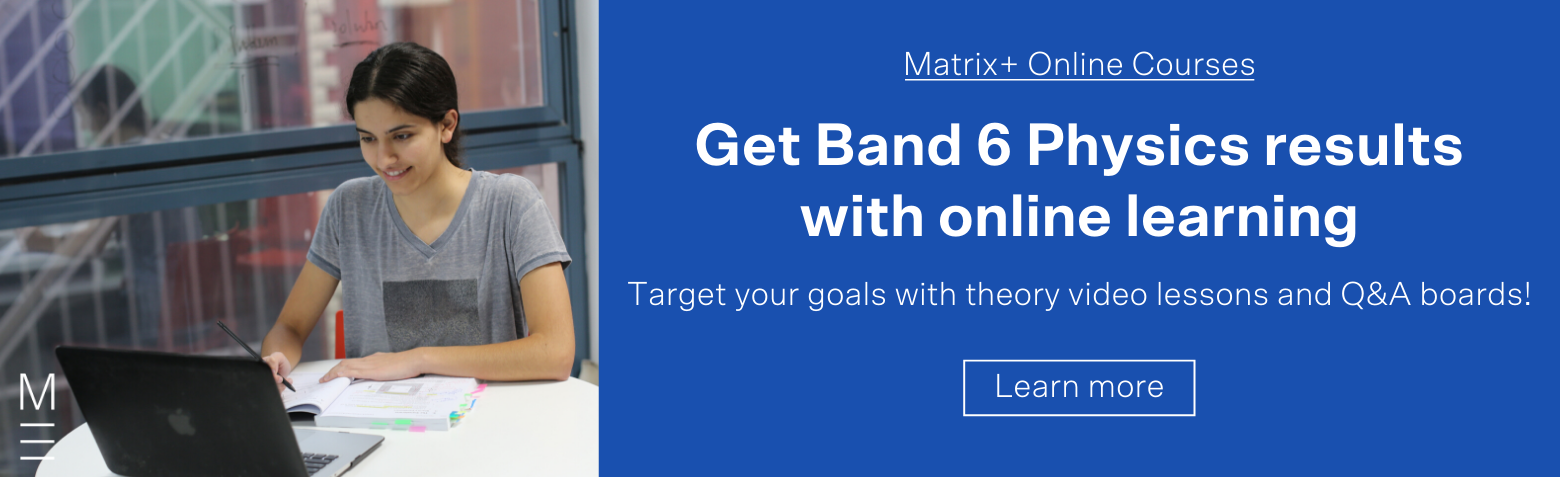# Advanced Mechanics | HSC Physics Study Guide Part 1

Send your Physics marks into orbit with this study guide!Are you struggling with Advanced Mechanics for HSC Physics? Don’t worry, in this article, we’ll explain the key concepts you need to know to fulfil the NESA Physics Outcomes.

## Advanced Mechanics | HSC Physics Study Guide Part 1

The HSC Physics Advanced Mechanics module contains a variety of fundamental and interesting concepts, focusing on gravity and how it affects motion. Part 1 of the HSC Physics study guide highlights some of these fundamental concepts using flowcharts to simplify your conceptual understanding.

At Matrix, students are taught to structure their answers using flowcharts to simplify complex processes.

## 1. Gravitational Fields and the Law of Universal Gravitation

The first and most important concept in the advanced mechanics module is the Law of Universal Gravitation. It describes the forces experienced by two masses as their gravitational fields interact. This occurs for any two objects that have mass, although in some circumstances the effect is too small to notice. Importantly, when two objects interact gravitationally, they each experience the same force. This is described by Newton’s Law of Universal Gravitation: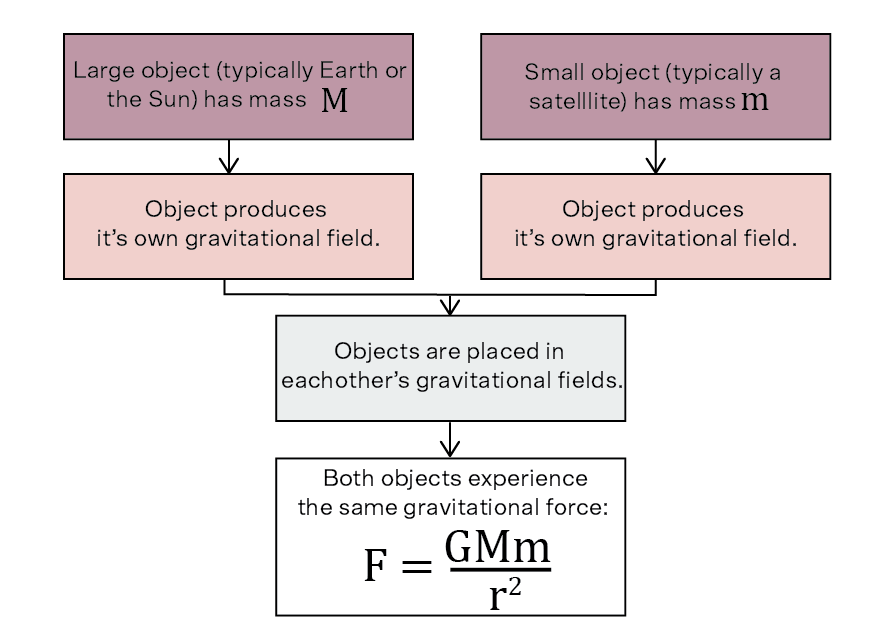## 2. Deriving equations based on Newton’s Law of Universal Gravitation

Several formulae important to Module 5 are derived from Newton’s Law of Universal Gravitation. Students may need to replicate (or conceptually explain) the derivation of some of these.  The following flowchart outlines the principal formulae derived from Newton’s Law of Universal Gravitation:

## 3. Uniform Circular Motion

Uniform circular motion is a phenomenon investigated briefly in Year 11. It returns as an important aspect of Advanced Mechanics, where we derive that if an object experiences a force that is constant in magnitude and always perpendicular to its velocity, it may undergo uniform circular motion: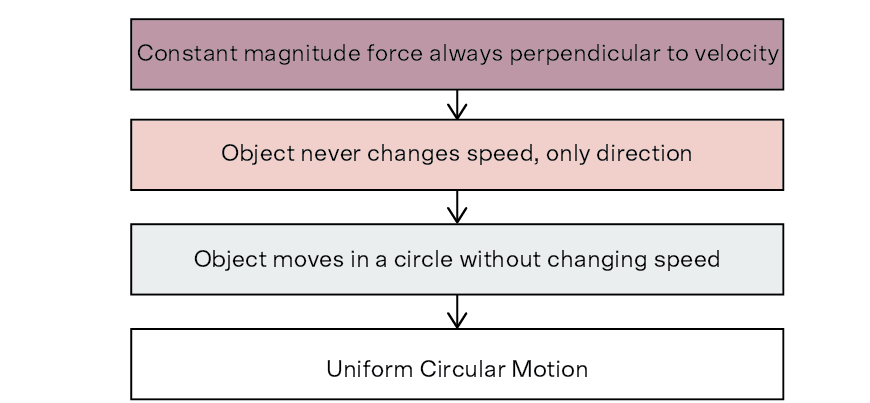## 4. Circular Orbits

Circular orbits are a special case of uniform circular motion where the gravitational force from the central object is providing the centripetal force. The requirements for a circular orbit are the same as that for normal uniform circular motion: a constant force that is always perpendicular to velocity. The following flowchart outlines the process for achieving a circular orbit: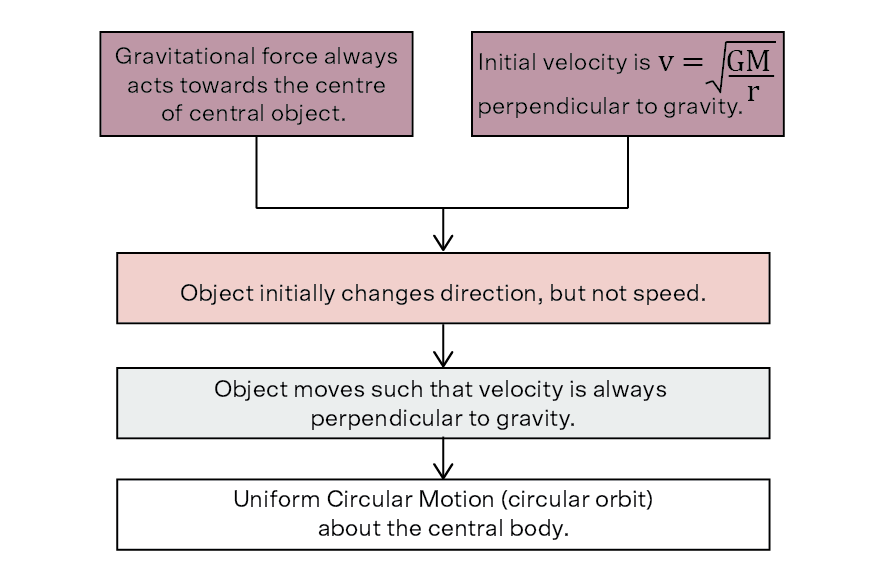## 5. Energy Changes in Orbits

One of the more poorly understood aspects of the Advanced Mechanics syllabus is the energy transformations that occur as an object changes between circular orbits of different radius. Two changes must be accounted for: the orbital velocity of the new orbit will be different (kinetic energy will change), and the orbital radius of the new orbit will be different (potential energy will change).  Therefore, the total energy of the two orbits is different and work must be done to change the object’s energy, such that it has the correct orbital speed and altitude for the new orbit. The flowchart below illustrates the changes that occur when an object moves from a larger radius orbit to a smaller radius orbit, compared to a simple change of altitude for a stationary object.

## 6. Kepler’s Second Law

Another difficult concept in Advanced Mechanics is Kepler’s Second Law, which describes objects in elliptical orbits. These objects travel faster when they are closer to their central body, and slower when they are further away. The following flowchart outlines this phenomenon via energy transformations: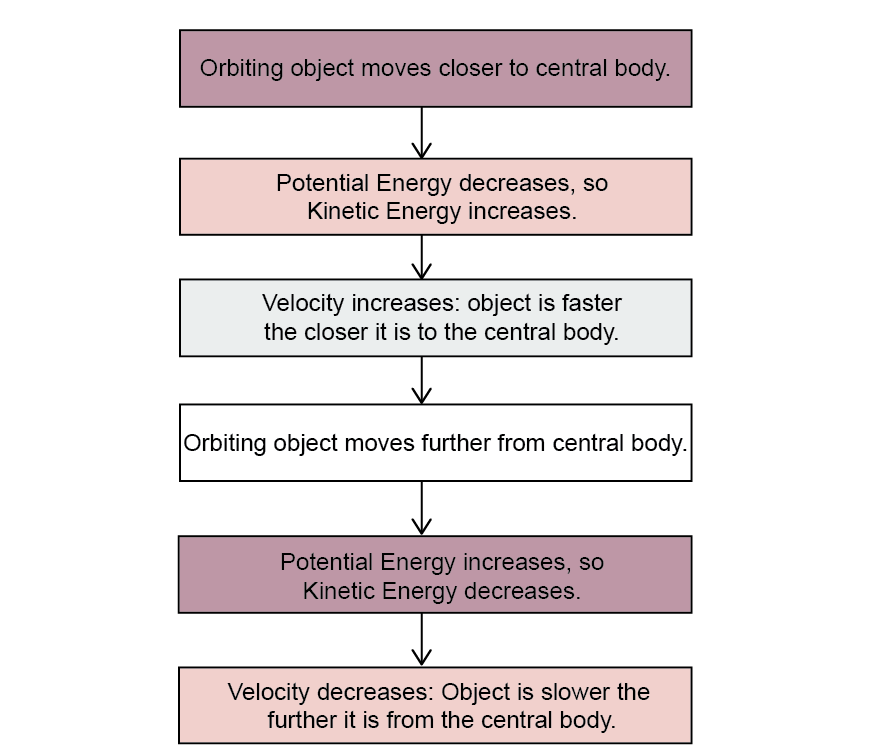## Looking for Part 2, Electromagnetism?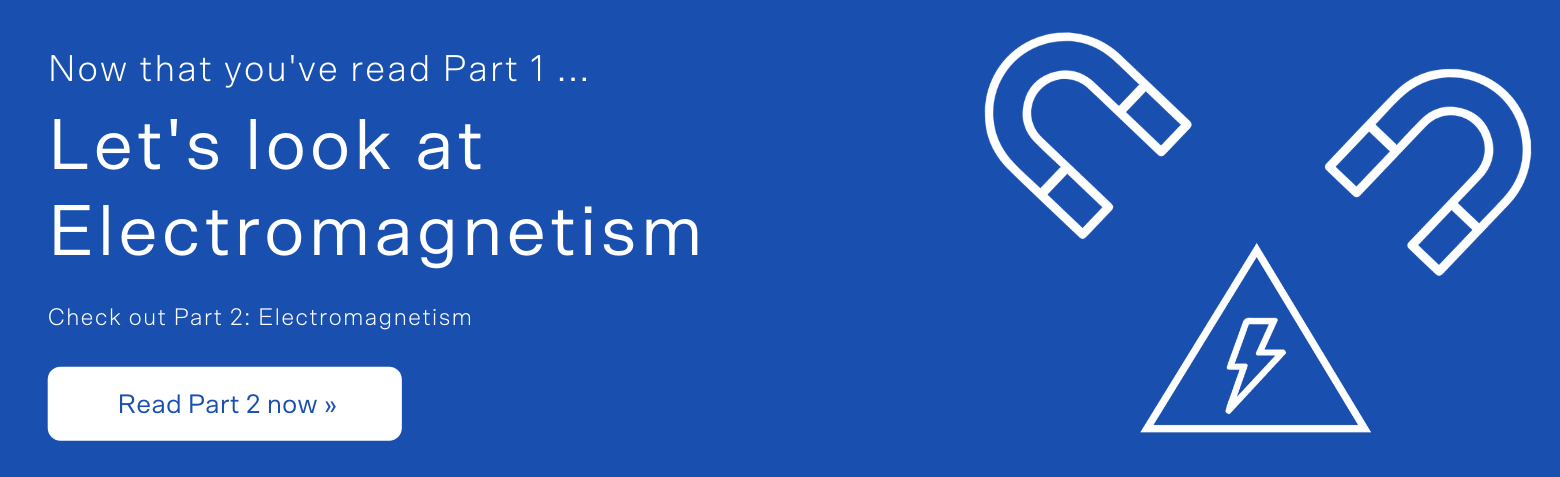## Want to Take Your Physics to the Next Level?

The Trial HSC Exams are around the corner! If you’re struggling with your Physics marks now, you’d better get on top of it before entropy really kicks in. Don’t worry, Matrix+ is here to help! Learn how to boost your HSC marks.